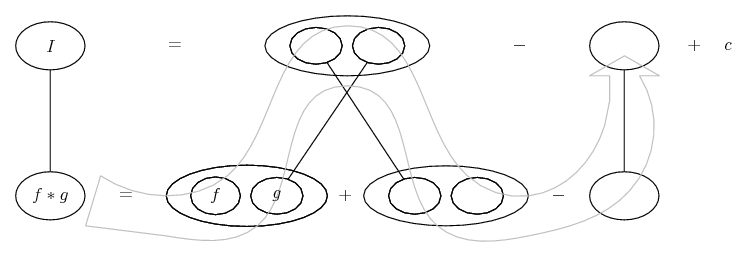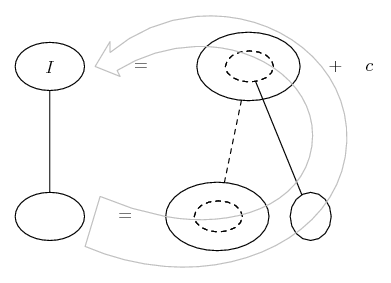# Thread: Indefinite integral

1. ## Indefinite integral

Compute:

Integral(2*x^3*e^(-x^2))
For some reason I make a mistake with the minus-sign, I don't see where. We substitute:

u = x^2
=>
du/dx = 2x
=>
dx = du/2x
Thus we can write the integral as:
Integral(u*e^(-u))
Using integration by parts yields:
-u * e^(-u) - (-1*e^(-u)) = (1-u)*e^(-u)
But my calculator says -(1+u) - what am I doing wrong?

2. ## Re: Indefinite integral

Only losing track. You have avoided using an integral sign, which hasn't helped. On the other hand, so have I...... where (key in spoiler) ...

Spoiler:... is the product rule, straight continuous lines differentiating downwards (integrating up) with respect to x.... is lazy integration by parts, doing without u and v.

Spoiler:Or, zooming in on the chain rule...

Spoiler:... where...

Spoiler:... is the chain rule. Straight continuous lines differentiate downwards (integrate up) with respect to the main variable (in this case ), and the straight dashed line similarly but with respect to the dashed balloon expression (the inner function of the composite which is subject to the chain rule).

The general drift is...I hope that does help.

Also, here it is with your sub...__________________________________________________ __________

Don't integrate - balloontegrate!

Balloon Calculus; standard integrals, derivatives and methods

Balloon Calculus Drawing with LaTeX and Asymptote!

#### Search Tags

indefinite, integral